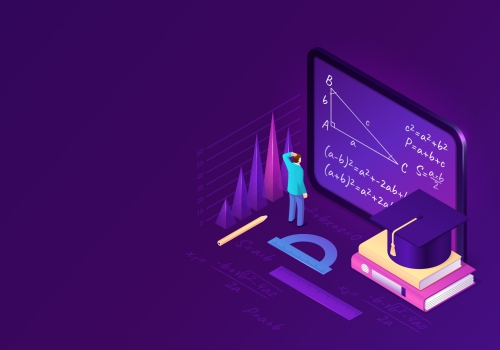×

# Mental Maths

Level - 01
• 150
Lectures
• 53:23:53
Duration

#### Course description

Mental computation requires students to quickly and efficiently recall a range of strategies and then select the best one for the problem at hand. Every new strategy learned and problem solved encourages brain plasticity – that is, increased brain-cell activity and the creation of new pathways.

Mental math is a group of skills that allow people to do math “in their head” without using pencil and paper or a calculator. One of these skills is remembering math facts, like 8 × 5 = 40. Other skills include rounding numbers and estimating calculations.

Mental math can also help kids understand math concepts better. Using and practicing mental math regularly helps kids improve their number sense .

For example, which is a better deal? Buying a box of 10 packs of gum for \$18.00 or buying individual packs for \$2.50 each? Use mental math to quickly estimate that the 10-pack must cost less than \$2.00 per pack because 10 × 2 = 20. This is a better deal than the second option where each pack costs more than \$2.00.

Mental math is useful in other ways too. It keeps math facts from getting rusty. It can also help get to the answer faster.

For example, what is 47 + 65? It’s easier to do this in your head if you break the numbers down into their digits’ values: 40 + 60 = 100 and 7 + 5 = 12. Add those two totals and you get the answer of 112.

Doing mental math requires strong memory skills. Math facts and other number concepts have to be retrieved from long-term memory. Our brains also use a skill called working memory to keep track of the steps needed to solve a particular math problem.

#### Tags

Mental Math, mathematics

#### Course content

• 20
CHAPTERS
• 75
Videos
• 75
PdfsCourse Price
₹500.00